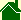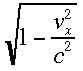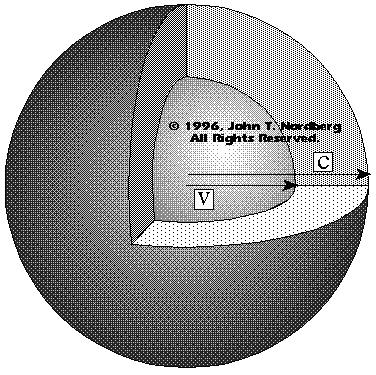#What is Time?

### Nordberg Interpretation

Time is a constant quantity of motion that we use to measure all other motions. Just as you use the constant length on a ruler to measure other lengths, you use a constant motion to measure other motions.

Through history, the motion we have used to measure other motions, the motion we have been calling "time" is the apparent motion of the sun crossing the sky for an earthbound observer.

John T. Nordberg defines time as: a constant quantity of motion, used to measure other motions, equivalent to what is now called the speed of light.

Imagine time as an expanding sphere of light.
Traditional physics has no accepted definition to the question, "What is time?"

Scientists think they know how to use time and how to measure time, but they do not think they know what time is!

What the does thismean?## Geometrical Interpretation of Einstein's Special Theory of Relativity

The above graphic provides a geometrical interpretation to Einstein's Special Theory of Relativity. I contend that Special Relativity is mathematical trick that solves the problem of using the sun's motion as our standard of motion. Looking at the above image, note the difference in the distance between arrow "c" and arrow "v." This distance is the error between using time defined at a sublight definition -- e.g., using the sun's motion -- and using time defined at the fastest known motion -- the speed of light.

If you are good with math and physics, you can derive Relativity from this geometry. (If you would like to see John Nordberg's derivation...)